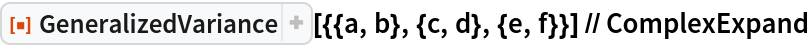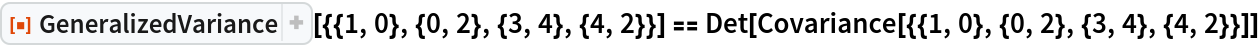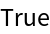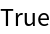Function Repository Resource:

# GeneralizedVariance

Find the determinant of the covariance matrix

Contributed by: Wolfram Research
 ResourceFunction["GeneralizedVariance"][matrix] gives the generalized variance for matrix.

## Details and Options

ResourceFunction["GeneralizedVariance"][matrix] effectively gives the determinant of the covariance matrix for matrix.
For numeric matrix, ResourceFunction["GeneralizedVariance"][matrix] is equal to Apply[Times,Variance[PrincipalComponents[matrix]]].

## Examples

### Basic Examples (1)

GeneralizedVariance of real-valued bivariate data:

 In:=Out=### Properties & Relations (2)

GeneralizedVariance is equivalent to the determinant of the covariance matrix:

 In:=Out=GeneralizedVariance is equal to the product of the principal component variances:

 In:=Out=## Requirements

Wolfram Language 11.3 (March 2018) or above

## Version History

• 1.0.0 – 20 February 2019